# AP Board 8th Class Maths Solutions Chapter 12 Factorisation InText Questions

## AP State Syllabus 8th Class Maths Solutions 12th Lesson Factorisation InText Questions

AP State Syllabus AP Board 8th Class Maths Solutions Chapter 12 Factorisation InText Questions and Answers.

### 8th Class Maths 12th Lesson Factorisation InText Questions and Answers

Do this

Question 1.
Express the given numbers in the form of product of primes. [Page No. 267]
(i) 48      (ii) 72      (iii) 96
(i) 48
48 = 2 × 2 × 2 × 2 × 3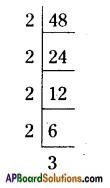ii) 72
72 = 2 × 2 × 2 × 3 × 3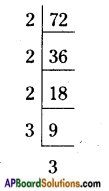iii) 96
96 = 2 × 2 × 2 × 2 × 2 × 3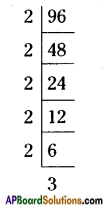Question 2.
Find the factors of following:      [Page No. 268]
(i) 8x2yz     (ii) 2xy (x + y)       (iii) 3x + y3z
i) 8x2yz = 2 × 2 × 2 × x × x × y × z
ii) 2xy (x + y) = 2 × x × y × (x + y)
iii) 3x + y3z = (3 × x) + (y × y × y × z)

Question 3.
Factorise:      [Page No. 270]
(i) 9a2 – 6a
(ii) 15a3b – 35ab3
(iii) 7lm – 21lmn
(i) 9a2 – 6a = 3 × 3 × a × a – 2 × 3 × a
= 3 × a (3a – 2)
∴ 9a2 – 6a = 3a (3a – 2)

ii) 15a3b – 35ab3
= 3 × 5 × a × a × a × b – 7 × 5 × a × b × b × b
= 5 × a × b [3 × a × a – 7 × b × b]
= 5ab [3a2 – 7b2]

iii) 7lm – 21lmn
= 7 × l × m7 × 3 × m × n × l
= 7 × l × m [1 – 3n]
= 7lm [1 – 3n]Question 4.
Factorise:
i) 5xy + 5x + 4y + 4
ii) 3ab + 3a + 2b + 2 [Pg. No. 271]
i) 5xy + 5x + 4y + 4
= (5xy + 5x) + (4y + 4)
= 5x(y + 1) + 4(y + 1)
= (y + 1) (5x + 4)

ii) 3ab + 3a + 2b + 2
= [3 × a × b + 3 × a] + [2 × b + 2]
= 3 × a [b + 1] + 2 [b + 1]
= (b + 1) (3a + 2)

Think, Discuss and Write

While solving some problems containing algebraic expressions in different operations, some students solved as given below. Gan you identify the errors made by them? Write correct answers.     [Page No. 279]
Question 1.
Srilekha solved the given equation as shown below.
3x + 4x + x + 2x = 90
9x = 90 Therefore x = 10 What could say about the correctness of the solution?
Can you identify where Srilekha has gone wrong?
Srilekha’s solution is wrong,
∵ 3x + 4x + x + 2x = 90
10x = 90
x = $$\frac{90}{10}$$
∴ x = 9

Question 2.
Abraham did the following.      [Page No. 280]
For x = -4, 7x = 7 – 4 = -3.
Abraham’s solution is wrong.
∴ If x = -4
⇒ 7x = 7(-4) = -28Question 3.
John and Reshma have done the multiplication of an algebraic expression by the following methods: verify whose multiplication is correct.      [Page No. 280]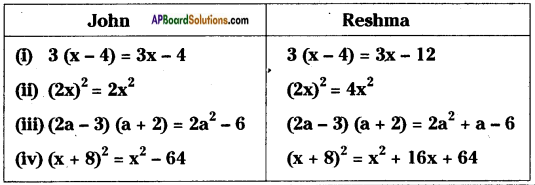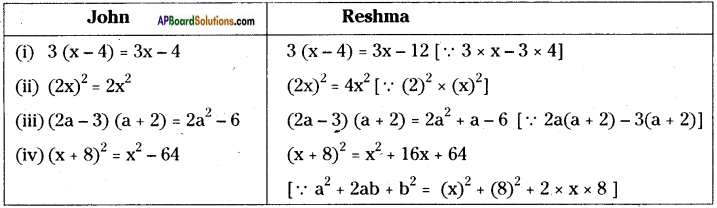(a + 5) ÷ 5 = $$\frac{a+5}{5}$$
= $$\frac{a}{5}$$ + $$\frac{5}{5}$$
= $$\left(\frac{a}{5}+1\right)$$